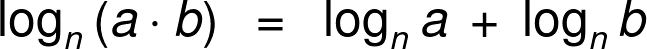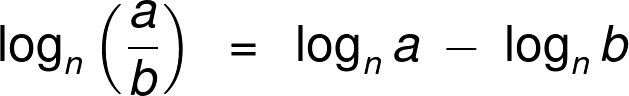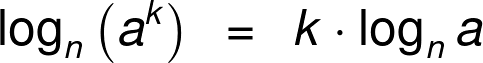# Expanding Logarithms Calculator

By Maciej Kowalski, PhD candidate
Last updated: Feb 08, 2021

Welcome to Omni's expanding logarithms calculator, where we'll learn to expand logarithmic expressions according to three simple formulas. The first one, the product property of logarithms, basically turns multiplication inside a log into adding logs. The formula for division works the same, but the sum changes into a difference. Lastly, the power property of logarithms allows us to take the exponent outside.

Arguably, the above description was vague. Hopefully, vague enough to inspire curiosity and willingness to see the log addition, difference, and exponent rules in detail and study how to expand the logarithmic expression together with our expand log calculator.

## What is a logarithm?

Some of you may disagree, but deep down, we all know that mathematics lies at the bottom of it all. From the very moment two rabbits met each other and decided to prolong the existence of their species, all they had in mind was mathematics, even if they didn't quite know it.

Apart from raising the probability of their survival (which, as we know, was very successful), the rabbits managed to become a symbol of multiplication. The meeting of two such animals (of the opposite sex, of course) leads to more rabbits. Therefore, if, for instance, we had 4 couples and each got 6 younglings, we describe the overall number of little ones using multiplication:

4 * 6 = 24

Obviously, the next generation has similar interests to their parents. Therefore, if each of a couple's kids got another 6 small ones later in life (with an outside partner, of course), and the same for their kids, and their kids, then we can calculate how big the fourth generation is with a new mathematical tool: exponents:

6⁴ = 1296

The logarithm is the inverse operation to an exponent. Our animal example corresponds to wondering how many generations have to pass before we get a fixed number of little ones. Therefore, for instance:

log₆(1296) = 4

Observe that the little ₆ corresponds to the size of a single litter. Note that taking a root is also considered an inverse operation to taking a power. However, as opposed to logarithms, roots return the exponent base, not the exponent itself (in the above language: they return the size of a single litter, not the number of generations that have to pass). For example:

⁴√1296 = 6

And for those who prefer symbols instead of bunnies, here's the formal definition of a logarithm:

 💡 logₐ(b) gives you the power to which you'd need to raise a in order to obtain b.

Before we expand the logarithmic expressions and see the formulas, let's mention a few essential facts concerning logs:

• There are two very special cases of the logarithm which have unique notation: the natural logarithm and the logarithm with base 10. We denote them ln(x) and log(x) (the second one simply without the small 10), and their bases are, respectively, the Euler number e and (surprise, surprise!) the number 10. There is also the binary logarithm, i.e., log with base 2, but it's not as common as the first two.
• The logarithm function is defined only for positive numbers. In other words, whenever we write logₐ(b), we require b to be positive.
• Whatever the base, the logarithm of 1 is equal to 0. After all, whatever we raise to power 0, we get 1.
• Logarithms are extremely important. And we mean EXTREMELY important. Outside of mathematics, they're used in statistics (e.g., the lognormal distribution), economics (e.g., the GDP index), medicine (e.g., the QUICKI index), and chemistry (e.g., the half-life decay). Also, quite a few physical units are based on logarithms, for instance, the Richter scale, the pH scale, and the dB scale.

Alright, we've come to know our new friend reasonably well. It's finally time to see how to expand logarithms, and we begin with the product property of logarithms, also known as the log addition rules.

## Product property of logarithms: log addition rules

The logarithm of a product is a sum of logarithms.The formula is a straightforward consequence of the definition of a logarithm and the rules for multiplying exponents.

Note how, a priori, when we expand the logarithmic expression of some number, we can choose any product we like. For example, we have:

log₅(100) = log₅(10 * 10) = log₅(25 * 4) = log₅(50 * 2),

and so according to the above product property of logarithms:

log₅(100) = log₅(10) + log₅(10) = log₅(25) + log₅(4) = log₅(50) + log₅(2).

Obviously, some choices are better than others. For instance, we have log₅(25) = 2, so we get one simple summand in the second decomposition. Nevertheless, it still leaves us with the second, uglier one.

Also, note that we can always work the formula backward and try condensing logarithms instead of expanding them. For instance, we can write:

log₆(9) + log₆(4) = log₆(9 * 4) = log₆(36) = 2.

The answer here turned out to be quite simple, although it was not at all clear beforehand.

With log addition rules behind us, we move on to subtraction. And the way we had multiplication in the prior, we'll now take division in the latter.

## Quotient property of logarithms: log difference rules

The logarithm of a quotient is a difference of logarithms.The formula is a straightforward consequence of the definition of a logarithm and the rules for dividing exponents.

Similar to the product property of logarithms in the above section, we can choose any quotient we like. For example, we have:

log₃(10) = log₃(20 / 2) = log₃(30 / 3) = log₃(100 / 10),

and so according to the above formula for quotient log expansion:

log₃(10) = log₃(20) - log₃(2) = log₃(30) - log₃(3) = log₃(100) - log₃(10).

Note how again it's best to think carefully about how we proceed. For instance, in the second decomposition, we have log₃(3) = 1, and we can work our way from there.

Moreover, we can again apply the formula the other way round and focus on condensing logarithms instead of expanding them. For instance, we can write:

log₄(128) / log₄(2) = log₄(128 / 2) = log₄(64) = 3.

Two down, one to go. Let's take on the last formula for today: the power property of logarithms, i.e., the log exponent rules.

## Power property of logarithms: log exponent rules

The logarithm of an exponent is a multiple of a logarithm.The formula is a straightforward consequence of the definition of a logarithm and the rules for taking a power of an exponent.

Again, we can choose the numbers in the formula to suit our purposes. However, while it's easy to see that 100 = 50 * 2 = 25 * 4, it's not so simple to describe one number as two different exponents and not use fractional powers. With some numbers, it's not that complicated, as we see in the example below:

log₇(64) = log₇(8²) = log₇(4³) = log₇(2⁶).

Applying the power property of logarithms, we get:

log₇(64) = 2 * log₇(8) = 3 * log₇(4) = 6 * log₇(2).

Note how the first two decompositions can be worked further. After all, 8 = 2³ and 4 = 2², so:

log₇(8) = log₇(2³) = 3 * log₇(2)

and

log₇(4) = log₇(2²) = 2 * log₇(2).

If we combine this with what we got above, both will give us what the third decomposition did from the start:

log₇(64) = 6 * log₇(2).

In other words, it seems best to use the log exponent rules with as high powers (and therefore, as small bases) as possible. Recall that prime factorization helps us establish just that.

Again, just like with the quotient and product properties of logarithms, we can have the formula work backward and use it for condensing logarithms instead of expanding them. For instance, we can write:

3 * log₃(9) = log₃(9³) = log₃(729) = 6.

Before we finish this section, let us mention one last thing. Namely, we'll explain how to get the quotient property from the log addition and exponent rules.

Recall that taking the -1 exponent of a number turns it into its multiplicative inverse. This means that, for example, 2⁻¹ = ½, (-3)⁻¹ = -⅓, or (⁴⁄₃)⁻¹ = ¾. We can use this fact to describe quotients using the -1 power and fraction multiplication:

2 / 3 = 2 * (1/3) = 2 * 3⁻¹,

1 / 16 = 1 * (1/16) = 1 * 16⁻¹,

- 2 / π = -2 * (1/π) = 2 * π⁻¹.

Therefore, we can apply that to logₙ(a / b), and then use the log addition and log exponent rules:

logₙ(a / b) = logₙ(a * (1/b)) = logₙ(a * b⁻¹) = logₙ(a) + logₙ(b⁻¹)

= logₙ(a) + (-1) * logₙ(b) = logₙ(a) - logₙ(b).

Voilà! We got the log expansion of the quotient. Pretty neat, wouldn't you say?

Now we leave the theory and move on to practice. It's time to see the expand log calculator in action!

## Example: using the expanding logarithms calculator

Suppose that while conducting some DIY experiment for your physics class, your calculations require you to find log₄(500). Clearly, it doesn't seem to be an integer, so we need to use some tricks to get at least closer to a reasonable approximation. And what better trick to use than log expansion?

Firstly, let's see how our expanding log calculator can help. There, we begin by picking one of the three options under "Use the formula for": product, quotient, or power property of logarithms. Let's try to expand the logarithmic expression we have as a sum of two others using the log addition rules (see the second section, so we choose logₙ(a * b).

We want to decompose log₄(500) so we input:

n = 4 and a * b = 500.

That gives the expanding logarithms calculator the product, but it still needs the factors to know what to do. Since our log is of base 4, why don't we pick that as one of them and input:

a = 4.

Note how that automatically computes b = 125 and returns the log expansion underneath step by step, together with the final value. However, before we reveal what that answer is, let's see how we can find it ourselves.

We have 500 = 4 * 125. According to the product property of logarithms, this gives:

log₄(500) = log₄(4 * 125) = log₄(4) + log₄(125) = 1 + log₄(125).

However, a keen eye may have noticed that 125 = 5³. Therefore, we can expand the logarithmic expression even further using the log exponent rules from the dedicated section:

log₄(500) = 1 + log₄(125) = 1 + log₄(5³) = 1 + 3 * log₄(5).

The last task is to find what log₄(5) is. We could try out some other nifty tricks like the change of base formula. After all, playing with logarithms proved to be quite some fun, right?

Right...?

Maciej Kowalski, PhD candidateUse the formula for
logₙ(a * b)
Variables
a
b
a * b
n
People also viewed…

### Circumference

Use this free circumference calculator to find the area, circumference and diameter of a circle.

### Linear interpolation

Our linear interpolation calculator allows you to find a point lying on a line determined by two other points.